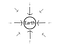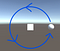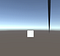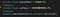# Simulating Gravity in Unity

Considering Unity is a game engine with built-in physics, it was not as easy as I thought it’d be.

Ok, let me clarify. If all you want to do is make a ball fall towards the ground, it’s incredibly easy. Add a rigid body, enable gravity, and you’re done.

The problem is it’s a simplified form of gravity. Like, Force of Gravity = Mass x Acceleration, where acceleration = 9.8m/s/s simple. This form, while useful for simulating gravity close to the Earth’s surface, is only useful for simulating gravity close to Earth’s surface.

It makes two assumptions:

1. The gravitational field is constant and downward
2. The acceleration is a constant 9.8m/s/s

However, as you move away from the earth, these assumptions don’t hold up, causing the approximation from this equation to become very inaccurate.

Away from the earth, gravity’s acceleration varies depending on where you are. Therefore, the gravitational field in the space around earth is much more complicated than just a downward Force=mass*9.8m/s/s. It has two key differences:

1. It points inward towards the earth
2. It decreases in strength as you move away from the earthAs you get farther from the earth, the force gets weaker

Due to these differences, to calculate the force of gravity, we need to use Newton’s law of universal gravitation.

Explained simply, this law states that the force of gravity between two objects is the mass of the first object (m1) times the mass of the second object (m2) times the gravitational constant (G) divided by the square of the distance between them (r).

Gravitational Constant = 6.67408 × 10^–11 m³ kg^-1 s^-2

If you want to simulate anything like orbital mechanics, this is the equation you need to use.

So let’s say we want to simulate orbital mechanics in Unity. We have a cube (let’s say this represents the sun) and we have a sphere (let’s say this represents a planet). Our goal is to have the planet orbit the sun in a proper ellipse.The sphere should orbit like this

If we enable gravity on the planet and sun, this is what happens:

It just pulls them both down… not exactly an orbit. Clearly, I have to write some code.

A good starting point is making a function to calculate the force of gravity.

First, I declared all the variables I need. These are primarily based on the variables in the equation for universal gravitation.

Next, I made a function to calculate the force of gravity.

This function is pretty simple. It’s just Newton’s law turned into code. First, I used the formula F=G*m*m / r² to calculate the magnitude of the force.

Then I used the difference of the sun’s position and planet’s position to give me the direction of the force. By multiplying this by the magnitude, I got the vector3 of the force that should be applied to the planet.

Now, I can call this function every time I need to calculate the force of gravity between the planet and the sun.

Before I make the planet orbit, it must have an initial velocity. I did this in the start function.

Next, because the force of gravity is continuously applied to an orbiting object, I need to calculate and apply it every frame.

To do this, it would be easiest to use the built-in rigidbody functions to manipulate the planet.

In the update function, I calculate the force of gravity and then add it to the planet with rigidbody.AddForce. This function should theoretically produce the desired result.

Here’s what happens:

Success! It’s moving in a circular orbit like motion, or that’s what I thought until I let it continue going. Unfortunately, here’s what the actual result was.

Yikes. That’s not even close to how an orbit should look. An object in orbit should always form an ellipse and return to the place it started. This method does not achieve that.

This is weird because, logically, the code should work, and it almost does, except it produces a strange path.

I eventually thought I discovered the problem. By default, to reduce the computational cost, Unity’s physics calculations have limited accuracy. So by using the built-in AddForce function, the reduced accuracy might cause this wacky mess.

One option to fix this is to go into the settings and force the physics calculations to be more accurate by changing the timestep.

Go to Edit -> Project Settings -> Time -> Fixed Timestep and change 0.02 to 0.002

This will force the FixedUpdate to run many more times each frame, resulting in better physics simulations. More times per frame = more calculations = higher accuracy.

To adjust to this change, the Update function also needs to be changed to FixedUpdate, and the force strength must be reduced by a factor of about 0.1 so that the planet is still able to orbit even though the force is being applied more frequently.

Because this is a simple computer simulation, the strength of the force varies with its magnitude and the number of times it’s applied per frame.

The old update function now looks like this:

And the results are:

This is definitely better, and it might even be good enough for some applications. However, it still has two problems.

Problem #1: It is not a perfect orbit.

Problem #2: Changing the Fixed Timestep changes it for the entire program. This will slow your program down. Depending on the size of your project, it might even make it unusable.

There was something wrong with my calculate force function.

Can you spot the error?

I forgot to normalize the direction of the force.

I calculated the direction using the difference between the position of the planet and the sun. The magnitude of this difference varies with their separation. Therefore, if they’re farther apart, the direction will have a higher magnitude.

That means, when I multiply the direction and force, the magnitude of the force is changed depending on the distance between the planet and sun.

To fix it, I have to normalize the difference to set its magnitude to one. This ensures the correct magnitude of the force from the initial calculation is maintained irrelevant of separation distance.

With this in mind, I fixed the function.

First, I added a line to calculate the heading (direction) of the force. This value always points towards the sun, just like gravity.

I then applied the magnitude of the force to the normalized value of the heading, giving the force the proper direction and magnitude.

Finally, after testing this code, I got proper orbits, although they seemed to glitch if the velocity was too high, so it’s important to be careful of that.

If you want to take advantage of the built-in Unity physics and rigidbody properties to do cool things like make the planet bounce off other objects, this is your best option. Even though the physics engine can sometimes be a bit tricky or glitchy, tweaking the numbers such as mass, velocity, and distance seemed to provide better results. However, if your main goal is an accurate orbital simulation, there is still another option⁠ — calculating the orbital path yourself!

This is, of course, more work than just letting Unity do the physics calculations, but once you finally get it working, I think it is a much more reliable solution.

Also, if you have difficulty making the other method work reliably, this is probably a better option.

If you took high school physics, maybe you recall learning about projectile motion or kinematics. There were many different equations, but by combining them and determining acceleration, force, and velocity, we can calculate the position of an object after a period of time.

To calculate the orbit of a planet, the simplest method I could think of is to calculate its motion for a very small timestep and then perform that calculation hundreds or thousands of times, until it makes a complete orbit.

First, I needed some new variables. They’re all pretty typical kinematics stuff.

Then I needed a new update function.

The code is mostly self-explanatory.

Here’s how it works:

• The time is set to 0.001seconds. This is how much “time” passes between each calculation. It’s a pretty random number. I only chose it because smaller ones seemed to have glitches and larger ones were still inaccurate.
• The original position of the planet is recorded.
• The acceleration is calculated using the old function for force and dividing by the planet’s mass. F=ma, therefore, a=F/m.
• The change in the position of the planet over 0.001 seconds is calculated.
• The planet’s position is moved by the change in position.
• The new position of the planet is recorded.
• Using the difference between the original and new positions divided by the time, the velocity of the planet is calculated. Recall v=d/t. The velocity is necessary for the change in position calculations.
• Before the velocity was calculated in the update function, an initial value was set in the start function. This initial value will affect the shape of the orbit. If the initial velocity is too large, the planet will have escape velocity and fly away. If the initial velocity is too small, the planet will accelerate into the sun. If the initial velocity is somewhere between, the planet will form a circular or elliptical orbit.

Here’s one of the results:Not supposed to happen

Yeah, not exactly what I wanted. At first, I thought it was because my initial velocity was too large, but, unfortunately, this is just a glitch that might happen when your numbers are too small, and they get rounded to zero. To fix it, I just used bigger numbers.Adding more zeros usually works

And the new result:

A perfect elliptical orbit! 🎉Some of the numbers don’t make a lot of sense (the masses and distances used were just random numbers that seemed to produce nice results), but the important thing is that the results make sense.

For me, it took a while to figure out all the glitches and understand why the objects weren’t behaving as they should. Hopefully, if you’re trying to build anything similar to this project, this article will help you avoid the challenges I faced.

Key takeaway: Sometimes, the code looks like it makes sense, but it just doesn’t work because of factors you don’t understand or can’t control. Use your problem-solving skills to figure out another way.